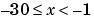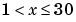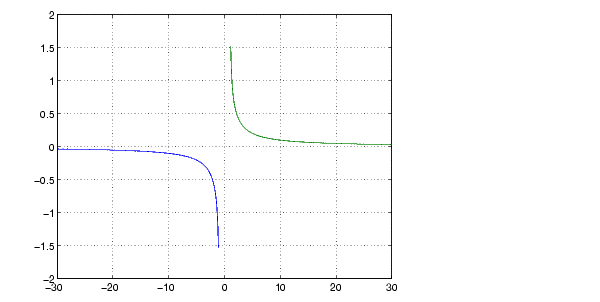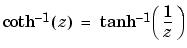MATLAB Function Referenceacoth

Inverse hyperbolic cotangent

Syntax

• ```Y = acoth(X)
```

Description

```Y = acoth(X) ``` returns the inverse hyperbolic cotangent for each element of `X`.

The `acoth` function operates element-wise on arrays. The function's domains and ranges include complex values. All angles are in radians.

Examples

Graph the inverse hyperbolic cotangent over the domains`` and.

• ```x1 = -30:0.1:-1.1;
x2 = 1.1:0.1:30;
plot(x1,acoth(x1),x2,acoth(x2)), grid on```

Definition

The hyperbolic inverse cotangent can be defined as

•Algorithm

`acoth` uses FDLIBM, which was developed at SunSoft, a Sun Microsystems, Inc. business, by Kwok C. Ng, and others. For information about FDLIBM, see http://www.netlib.org.

See Also

`acot`, `coth`

© 1994-2005 The MathWorks, Inc.